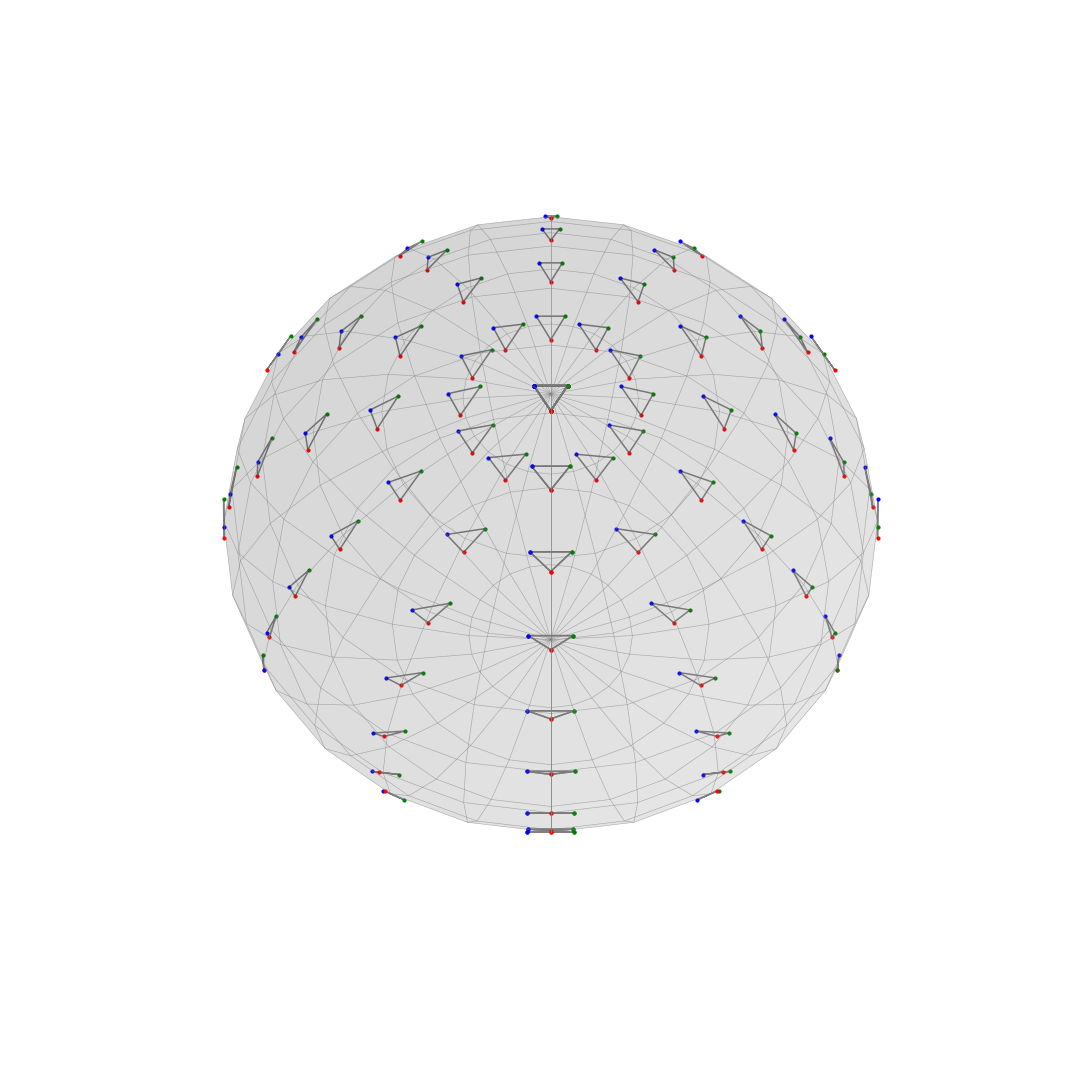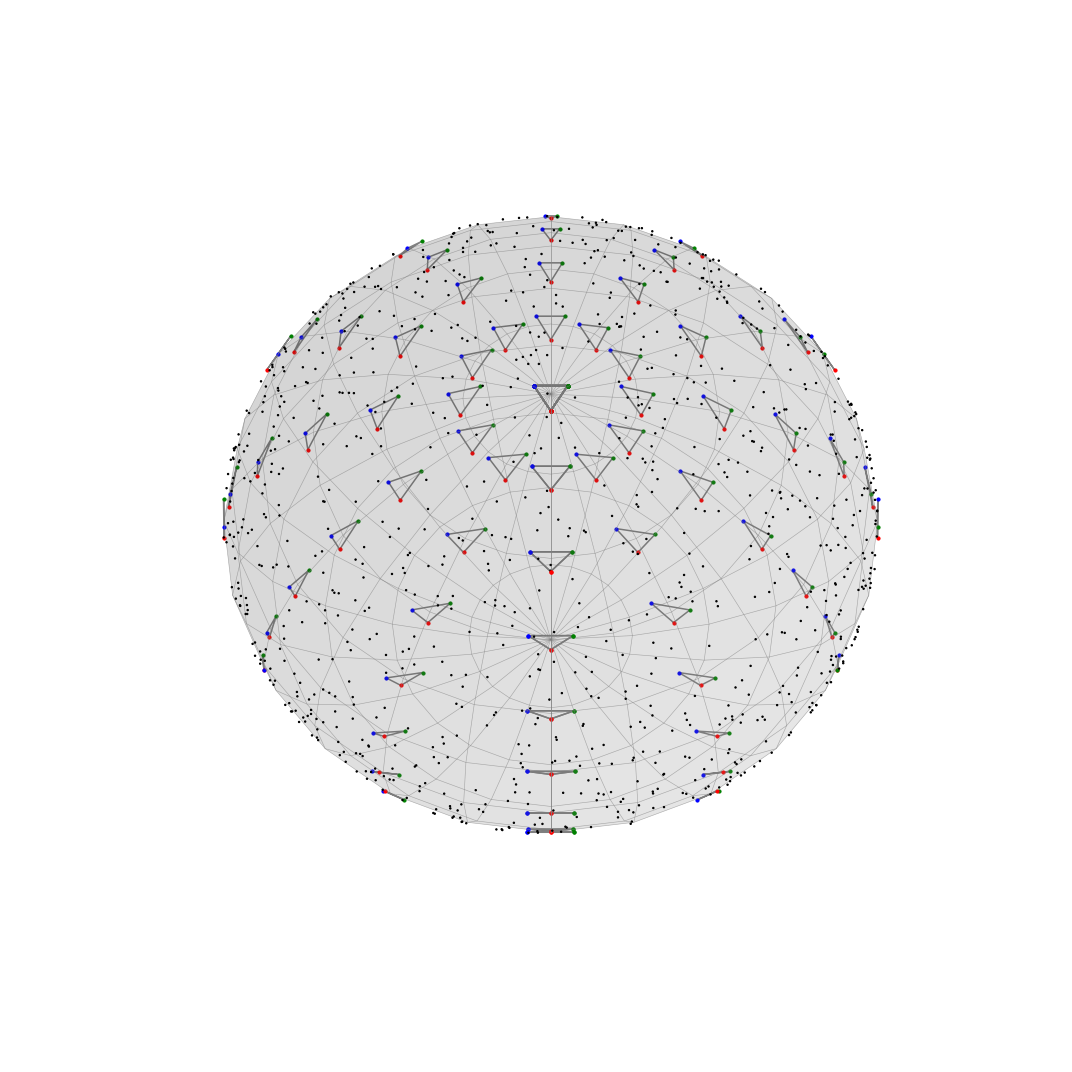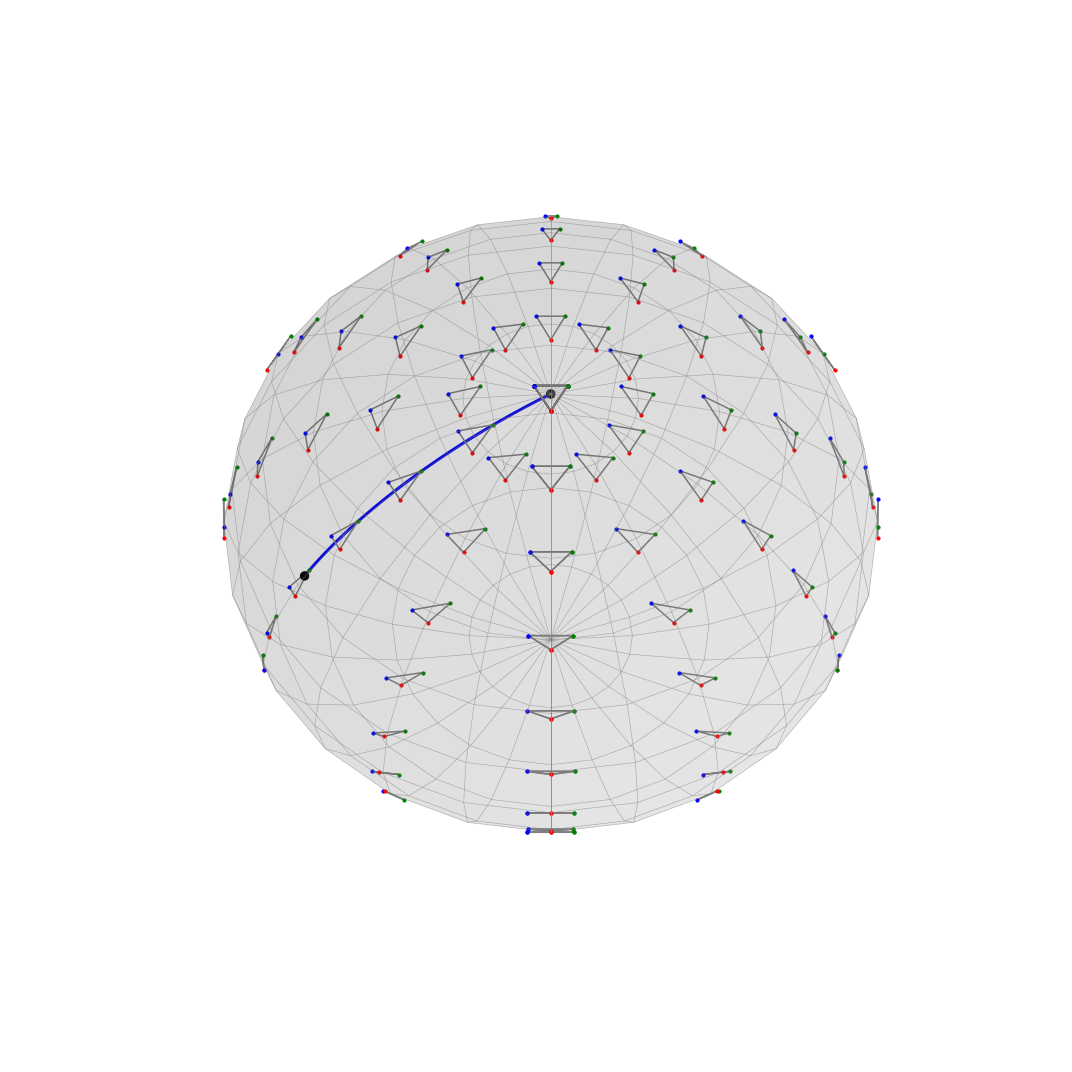# Computing with triangular shapes in Kendall framework¶

David G. Kendall first took interest into triangular shapes while studying random alignments of points in archeology and related fields [KK1980]. Following this statistical study, he developped the more general theory of shapes. This theory echoes in many fields like biology, medicine, or image analysis, where the shape of an object is particularly relevant when it comes to its analysis. Since the deformation of a shape is not necessarely linear, we need to use geometric statistics in order to proceed with such data.

In Kendall theory, the word shape is used in the common sense, refering to the appearance of an object. Since the shape of an object should not depend on the way it is looked at or computed, it is natural to remove location, rotationnal and eventually scale effects. A practical way of describing an object's shape is to locate a finite number of relevant points on it, which we call landmarks, which should summarize the geometrical and/or scientifical information [DM2016].

Thus we define Kendall shape space of order $(k,m)$ (where $k\geq 2$) as the quotient of $k$-tuples of non-aligned points in $\mathbb{R}^m$ by the group of rotations, translations and dilatations of $\mathbb{R}^m$ :

$$\big(\Sigma^k_m\triangleq\mathbb{R}^{km}\setminus \Delta \big)/\big(\mathbb{R_+^*}\times SO(m)\ltimes \mathbb{R}^m\big)$$

where $\Delta =\{\ (a)_{1\leq i\leq k}\ |\ a\in\mathbb{R}^m\}$ correspond to the degenerate case where all landmarks collapse into a unique point.

It is useful to see that considering first the action of translations and dilatations before applying rotation group $\text{SO}$ allows to write :

$$\Sigma^k_m=\mathcal{S}^k_m/ \text{SO}(m)$$

where $\mathcal{S}^k_m$, called the pre-shape space of order $(k,m)$ denotes the space of centered and normalized $k$-tuples of in $\mathbb{R}^m$. It corresponds to the unit sphere of dimension $k(m-1)$ [K1984].

The Kendall shape space of order $(k,m)$ is a differential manifold which acquires singularities as soon as $m\geq 3$. Apart from these singularities, it is a smooth manifold which can be equipped with a Riemannian metric inherited from the Frobenius metric on the pre-shape space [LK1993].

In this tutorial, we take a deeper look at two specific cases of Kendall shape spaces : the space $\Sigma^3_2$ of 2D triangles and the space $\Sigma^3_3$ of 3D triangles. These two spaces are particularly interesting to look at because there exists a projection such that we can directly visualize them.

The tutorial is organized as follows :

• First, we present the case of 2D triangles, and illustrate some geometric tools with a dedicated visualization class.
• Then, following the same scheme, we look at the 3D case.
• Finally, we explore these visualizations with the dataset of the optical nerves head

## Set up¶

In :
import os
import sys
import warnings

sys.path.append(os.path.dirname(os.getcwd()))
warnings.filterwarnings("ignore")

In :
%matplotlib inline

import matplotlib.pyplot as plt
from mpl_toolkits.mplot3d import Axes3D

import geomstats.backend as gs
import geomstats.datasets.utils as data_utils
from geomstats.geometry.pre_shape import KendallShapeMetric, PreShapeSpace
from geomstats.visualization import KendallDisk, KendallSphere

INFO: Using numpy backend


## The space $\Sigma_2^3$ of 2D triangles¶

In :
m_ambient = 2
k_landmarks = 3
preshape_triangle_2d = PreShapeSpace(3, 2)
metric_triangle_2d = KendallShapeMetric(3, 2)


The space $\Sigma_2^3$ of 2D triangles is a manifold of dimension $2=3\times(2-1)-1$. Kendall showed that it is isometric to the $2$-sphere of radius $1/2$ [K1984]. Such an isometry can be computed explicitly. Practically, this means that 2D triangular shape datasets can be directly visualized in 3D without losing any information (especially geometrical ones). Furthermore, all geometric statistics can be directly processed on the sphere, for which we already have a large catalogue of explicit methods.

First step consist in projecting (via the isometry mentionned above) the triangles on the sphere. In order to visualize better how the space $\Sigma_2^3$ is mapped onto the sphere $S^2(1/2)$, we plot regularly sampled triangles at their corresponding location (according to the projection).

In :
S = KendallSphere()

In :
fig = plt.figure(figsize=(15, 15))
S.draw()One can easily notice that the equator corresponds to flat triangles while first meridian is paired with the subset of isocele triangles whose apex is red.

We now illustrate how some essential statistic and geometric tools project on the sphere.

## Uniform distribution¶

Since the projection is isometric, the uniform distribution in $\Sigma^3_2$ is exactly the uniform distribution on the sphere.

In :
fig = plt.figure(figsize=(15, 15))
S.draw()

points = preshape_triangle_2d.random_uniform(1000)

S.clear_points()
S.draw_points(color="k", s=2)## Geodesic¶

The geodesics in $\Sigma^3_2$ corresponds to great circles on the sphere. The distance between two triangles of $\Sigma^3_2$ is given exactly by the arc-length of the arc joining the two projected points on the sphere. The diameter of the space $\Sigma^3_2$, defined as the distance between the two most distant points, is then equal to $\pi/2$.

In :
fig = plt.figure(figsize=(15, 15))
S.draw()

times = gs.linspace(0.0, 1.0, 1000)
velocities = gs.array([-t * 0.5 * S.ub for t in times])
points = metric_triangle_2d.exp(velocities, S.pole)

S.clear_points()
S.draw_curve(color="b", lw=3)
S.clear_points()
S.draw_points(color="k", s=70)## Parallel transport¶

Given the geodesics and the logarithm map, one can easily compute the parallel transport of a vector $w$ along the geodesic $\exp_\text{b.p.}(tv)$ using the Pole Ladder algorithm implemented in geomstats.

In :
base_point, v, w = S.pole, -0.5 * S.ub, S.ua
n_rungs = 10
w, base_point, v, n_rungs, return_geodesics=True
)

n_points = 10
t = gs.linspace(0.0, 1.0, n_points)
t_main = gs.linspace(0.0, 1.0, n_points * 4)
main = []
diag = []
for points in trajectory:
main_geodesic, diagonal, final_geodesic = points
main.append(main_geodesic(t_main))
diag.append(diagonal(-t))
diag.append(diagonal(t))

diag = gs.stack(diag).reshape(-1, k_landmarks, m_ambient)
main = gs.stack(main).reshape(-1, k_landmarks, m_ambient)

tangent_vectors = [v / n_rungs, w / n_rungs, transported / n_rungs]
base_points = [base_point, base_point, main[-1]]

In :
fig = plt.figure(figsize=(15, 15))
S.draw()

S.clear_points()